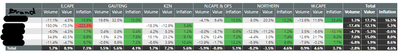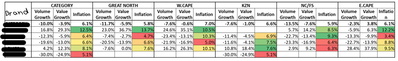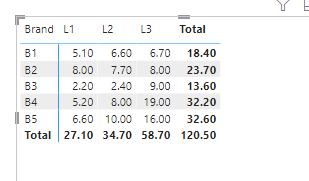cancel
Showing results for
Did you mean:Frequent Visitor

## Conditional Formatting in Matrix with Measures Grouped by Columns

Hi All,

Hope you can help.

I am trying to wrap my head around Conditional formatting using a gradient for a Matrix that has Columns based off Measures.

The gradient conditional formatting is working, but it is ignoring the filter context of the Columns. It is setting the gradient over all values for the Provinces (See Below), I would imagine this is exactly what is needed in most cases. But I am wanting to achieve a gradient conditional formatting per Province for the Inflation Measure.

Current Result:Expected Result:In the expected result, there is a conditional formatting Gradient happening for each Inflation Column By province.

Not sure this is possible to achieve, but your input would be greatly appreciated.

Let me know if there is any further info I should supply.

Thanks again.

1 ACCEPTED SOLUTIONCommunity Support

Hi , @Slabbos

If you want to show the two color you can try to add some judgement in dax code , like this:

``````Measure 2 =
var _rankx = rankx(ALL('Table'[Brand]),CALCULATE( SUM('Table'[Value])),,DESC,Dense)
var init_color = 0.5
var _end_color =FORMAT( ((1-init_color)/(COUNTROWS(ALLSELECTED('Table'[Brand]))/2) )*_rankx , "0%")
var _end_color2 =FORMAT((COUNTROWS(ALLSELECTED('Table'[Brand]))-_rankx)*(init_color/(COUNTROWS(ALLSELECTED('Table'[Brand]))/2))+init_color,"0%")

return
IF(_rankx< COUNTROWS(ALLSELECTED('Table'[Brand]))/2 , "hsl(80,100%,"&_end_color&")",   "hsl(0,100%,"&_end_color&")")
``````

Then we can get this:Thank you for your time and sharing, and thank you for your support and understanding of PowerBI!

Best Regards,

Aniya Zhang

If this post helps, then please consider Accept it as the solution to help the other members find it more quickly

5 REPLIES 5Frequent Visitor

Thank you very much for the reply. Will have a look at your solution and let you know how it goes.Community Support

Hi ,  @Slabbos

According to your descripition, you want to "Conditional Formatting in Matrix with Measures Grouped by Columns".

I tested it in my side, and the built-in gradient conditional format cannot take effect according to the context. He judges all the fields that are put in.

For your need , you can try to use the dax code to try to realize it .

Here are the steps you can refer to :
(1)This is my test data:(2)Then we can create a measure like this:

``````Measure =
var _rankx = rankx(ALL('Table'[Brand]),CALCULATE( SUM('Table'[Value])),,DESC,Dense)
var init_color = 0.5
var _end_color =FORMAT( init_color + (1-init_color)/COUNTROWS(ALLSELECTED('Table'[Brand]))*_rankx,"0%")
return
"hsl(220,100%,"&_end_color&")"``````

(3)We can configure it in the background color:

The result is as follows:Thank you for your time and sharing, and thank you for your support and understanding of PowerBI!

Best Regards,

Aniya Zhang

If this post helps, then please consider Accept it as the solution to help the other members find it more quicklyFrequent Visitor

Hi,

Thank You, I get the Idea, and I may be able to work with this. But it seems your solution is for 1 colour only. I would like to have it be a gradient from Green(Highest Value) to Red(Lowest Value).

Will play around and let you know if I can figure it out.Community Support

Hi , @Slabbos

If you want to show the two color you can try to add some judgement in dax code , like this:

``````Measure 2 =
var _rankx = rankx(ALL('Table'[Brand]),CALCULATE( SUM('Table'[Value])),,DESC,Dense)
var init_color = 0.5
var _end_color =FORMAT( ((1-init_color)/(COUNTROWS(ALLSELECTED('Table'[Brand]))/2) )*_rankx , "0%")
var _end_color2 =FORMAT((COUNTROWS(ALLSELECTED('Table'[Brand]))-_rankx)*(init_color/(COUNTROWS(ALLSELECTED('Table'[Brand]))/2))+init_color,"0%")

return
IF(_rankx< COUNTROWS(ALLSELECTED('Table'[Brand]))/2 , "hsl(80,100%,"&_end_color&")",   "hsl(0,100%,"&_end_color&")")
``````

Then we can get this:Thank you for your time and sharing, and thank you for your support and understanding of PowerBI!

Best Regards,

Aniya Zhang

If this post helps, then please consider Accept it as the solution to help the other members find it more quicklyFrequent Visitor

Hi,

Thank You. That is it. Just 1 Slight change, you didnt use _end_color2. After changing that it works perfectly. Thanks again for your help.

``````Measure 4 =
var _rankx = rankx(ALL('Table'[Brand]),CALCULATE( SUM('Table'[Value])),,DESC,Dense)
var init_color = 0.5
var _end_color =FORMAT( ((1-init_color)/(COUNTROWS(ALLSELECTED('Table'[Brand]))/2) )*_rankx , "0%")
var _end_color2 =FORMAT((COUNTROWS(ALLSELECTED('Table'[Brand]))-_rankx)*(init_color/(COUNTROWS(ALLSELECTED('Table'[Brand]))/2))+init_color,"0%")

return
IF(_rankx< COUNTROWS(ALLSELECTED('Table'[Brand]))/2 , "hsl(80,100%,"&_end_color&")",   "hsl(0,100%,"&_end_color2&")")``````